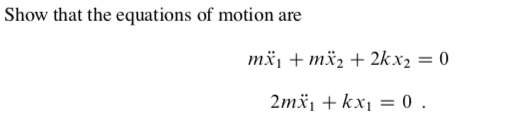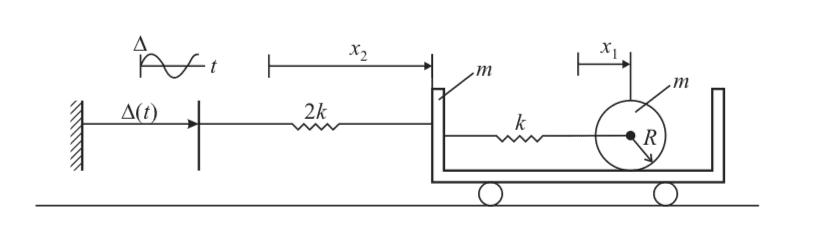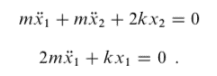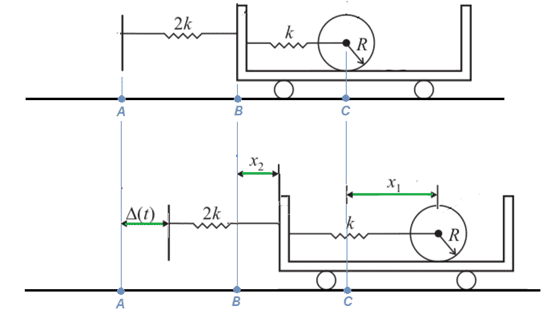# Derivation of the Equations of Motion for a System

Summary:: This is a system and we want to find the equations of motion. After some force-based attempts, I think that it would be easier to use some energy methods.

Hi,

I wanted to ask about deriving equations of motion by using the Lagrangian. The question is in the picture below. We are also told: 'The trolley is itself tied by a spring of stiffness 2k to a plate which is subject to harmonic motion. The roller does not slip.'

We have never been taught about the Lagrangian in our course (other than a mention that 'energy methods exist'), but I feel that I am wasting a lot of time trying to attempt this system with a force based approach. (there is no solution is available for this question)

I have never used the Lagrangian methods before, so I was just wondering whether I could check the terms that I am setting up within my Lagrangian? (I should be able to do the differentiation from there)My attempt:
From what I understand, the Lagrangian is $L = T - V$ and then I will differentiate with respect to the different variables to get the two equations.
For the kinetic energies, I have: $$T = KE_{cart} + KE_{mass translation} + KE_{mass rotation}$$
$$T = \frac{1}{2} m \dot x_2 ^2 + \frac{1}{2} m \left(\dot x_1 + \dot x_2 \right) ^2 + \frac{1}{2} \left( \frac{mR^2}{2} \right) \left( \frac{\dot x_1 + \dot x_2}{R} \right) ^ 2$$

For the potential energies, I have:
$$V = EPE_{2kspring} + EPE_{kspring} = \frac{1}{2} (2k) \left( x_2 - \Delta \right)^2 + \frac{1}{2} (k) \left( x_1 - x_2 \right)^2$$

Looking at these, these don't look correct to me, although I am struggling to understand what I have done wrong. Should I have included the $\Delta$ term in the Kinetic energy expressions? I would appreciate any guidance on this new topic for me. Thanks in advance.

Please note, I did try to look online, but I found lots of very simple examples that were of different systems/ extremely simple systems, so those weren't of much help to me.

## Answers and Replies

TSny
Homework Helper
Gold Member
Are ##x_1## and ##x_2## measured from fixed positions on earth?

If so, then I get the equations of motion listed in your post except that I also get a term in each equation that involves ##\Delta##.

Are ##x_1## and ##x_2## measured from fixed positions on earth?

If so, then I get the equations of motion listed in your post except that I also get a term in each equation that involves ##\Delta##.

Thank you for your reply. It doesn't say how the coordinates are defined. Actually, I think they might be defined intrinsically (at least I will try that first as it is the easier of the two cases).

I think I may have found a sensible force-based approach whereby I introduce an intermediary 'frictional' type force between the roller and the cart. Then I can resolve horizontally for both of the bodies and take moments about the COM of the roller to get the third equation to help get rid of the force.

TSny
Homework Helper
Gold Member
It doesn't say how the coordinates are defined. Actually, I think they might be defined intrinsically (at least I will try that first as it is the easier of the two cases).

You gave the answer for the equations of motion asI get this result except that I find that each equation has an additional term involving ##\Delta (t)##. It seems to me that ##\Delta(t)## should enter the equations of motion.

I chose the definitions of ##x_1## and ##x_2## as indicated belowThe top figure shows the system in the special configuration where the plate is at ##\Delta = 0## and both springs are at their natural length. Point ##A## is a fixed point on the ground below the ##\Delta = 0## position. Point ##B## is a fixed point on the ground below the left end of the cart in this figure, and point ##C## is a fixed point on the ground below the center of the cylinder in this figure.

The bottom figure shows a general configuration of the system where the plate has moved to ##\Delta(t)## and the springs could be stretched or compressed. I defined ##x_1## to be the horizontal displacement of the center of the cylinder from the fixed point ##C## and I defined ##x_2## to be the horizontal displacement of the left end of the cart from the fixed point ##B##.

I think I may have found a sensible force-based approach whereby I introduce an intermediary 'frictional' type force between the roller and the cart. Then I can resolve horizontally for both of the bodies and take moments about the COM of the roller to get the third equation to help get rid of the force.
Yes, that sounds good. I found that it takes about the same amount of work to get to the equations of motion using the Lagrangian method or using the force-based method. The Lagrangian method is kind of elegant and you don't need to worry about the friction force between the cylinder and the floor of the cart.

•Master1022
The Lagrange formulation includes a generalized force term. This seems to be missing here.

The Lagrange formulation includes a generalized force term. This seems to be missing here.
Would this term arise from the excitation from the moving plate on the left?

Sure, the moving plate has to enter the problem in some way.